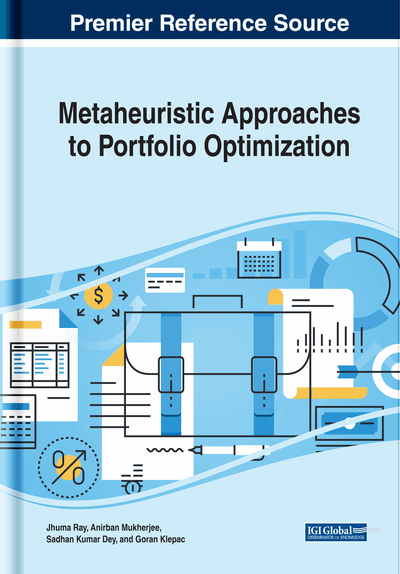# Bayesian Networks and Evolutionary Algorithms as a Tool for Portfolio Simulation and Optimization

Goran Klepac (Algebra University College, Croatia & Raiffeisen Bank Austria d.d., Croatia) and Leo Mrsic (Algebra University College, Croatia)
DOI: 10.4018/978-1-5225-8103-1.ch003
Available
\$37.50
No Current Special Offers

## Abstract

This chapter will propose solution how to recognise important factors within portfolio, how to derive new information from existing data and evaluate its importance factor. The chapter will also propose methodology for sensitivity evaluation between factors recognised as important. This information has valuable factors for Bayesian network construction. Such created Bayesian network can be used as simulation tool, as well as tool for portfolio optimization. As a simulation tool, such Bayesian network can be for output analysis regarding potential decisions via decision graphs. Also, as an optimization tool, Bayesian network can be used in way of finding optimal value of decision factors upon expected outputs from portfolio. For achieving this aim, evolutionary algorithms will be used as optimization tool.
Chapter Preview
Top

## Introduction

Bayesian networks provide optimization in which the presumption of attribute independence is maintained, but with the exception of where it is usable in terms of final outcome efficiency. Exceptions are variables, i.e. sets of variables, which are declared dependent, and this dependence is defined by a common distribution of probability for this set. Bayesian network shows a common distribution of probable outcomes for attributes dataset presented by a collection of assumptions on conditional dependencies and sets of local conditional probabilities. Bayesian belief network hierarchy could be designed in different way using algorithm or node training, but from the business, value and general performance perspective, it is adviseable that Bayesian ruleset should be improved by industry experts. Bayesian logic is often used in probability space, due to its capabilities and fact that their structure often depends on industry expert knowledge. The Bayesian network can be used to evaluate the value of a target variable with the values given by other variables. In the general case, it will be necessary to estimate the probability distribution of any subset of network variables by the given values or the distribution of any subset of the remaining variables. The exact calculation of these probabilities in the general Bayes network is NPs difficult. Therefore, many methods have been developed that sacrifice precision for efficiency. In theory, even approximation of probability in Bayes' networks may be NP hard. But in practice, such methods have proved usable in many cases.

### Learning Bayesian Network

There are several key concepts to be considered while in learning Bayesian networks: is the network paths known in advance, does the strucute must be learned, do any variables in each instance of learning really learn and are some instances may be invisible. The simplest case is, of course, learning the network if it is the default structure and if all the variables are always visible. Then we can apply a direct method, ie to estimate probability probability tables in average learning values, as in the Simple Bayesian Classifier. If, on the other hand, some variables are not visible the problem is similar to the problem of learning neural networks. One of the options is a gradual climbing technique in the space of a hypothesis corresponding to all the possible values in the probability probability tables. The objective function that is then maximized is the likelihood, the probability of the realization of the observed set with the condition of the hypothesis. Learning of Bayesian networks when the network structure is not known in advance is also difficult. There are developed metrics for dial-up between alternative networks and a heuristic search algorithm that learns the structure when the data is fully visible.

By participating in network structure, industry experts can not be a guarantee that the final network will be optimally designed but it will however be consistent with business expectations and environment. This chapter will present best practice taken from various projects combined into proposal for future hypothesize definition with goal to improve model robustness by using patters extension within the belief/probability network not only before but after network structure once take place. These actions have foundations in gain score for every node along the network, especially ones observed. Chapter methodology challenge expert knowledge on several levels with aim to to improve efficiency as well as to improve quality of hypothesis looking into impacts between nodes (impacts between nodes, objects and/or business valuation). As a result of such approach, final network recognizes greater predictive value, greater impact stability and improved robustness.

## Complete Chapter List

Search this Book:
Reset